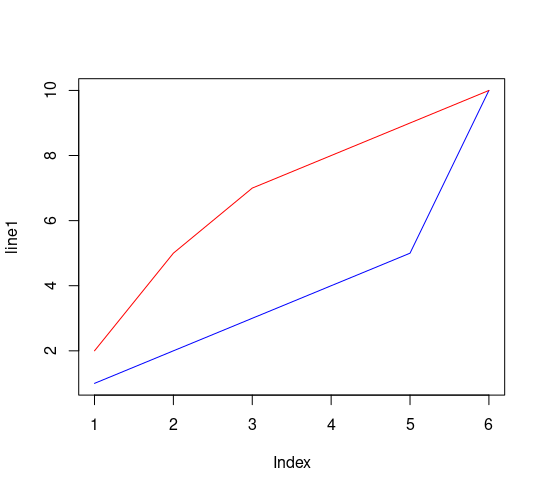# R 语言 – 绘制折线图

## 折线图

plot(1:10, type="l")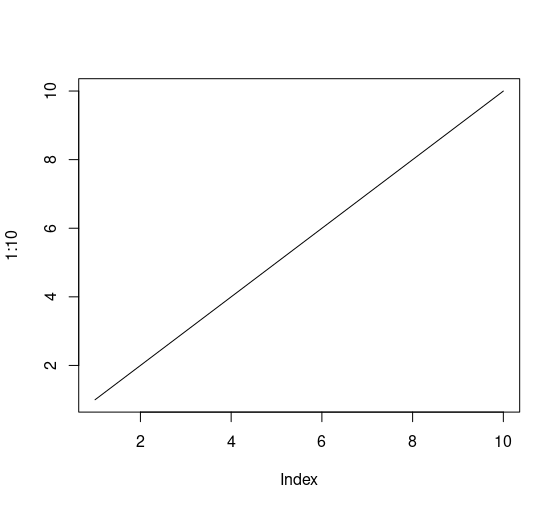## 线条颜色

plot(1:10, type="l", col="blue")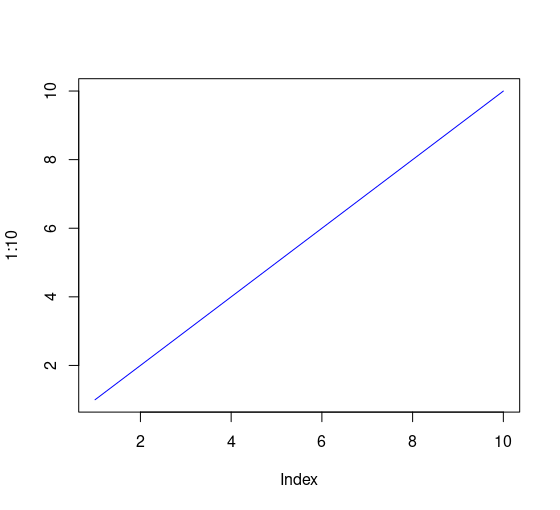## 线条宽度

plot(1:10, type="l", col="blue", lwd=2)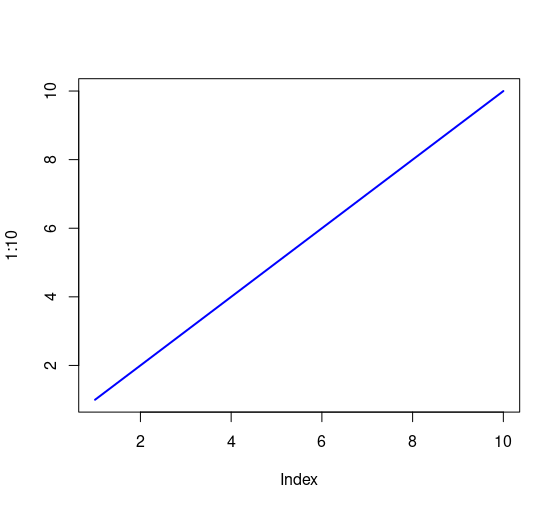## 线条样式

plot(1:10, type="l", col="blue", lwd=5, lty=3)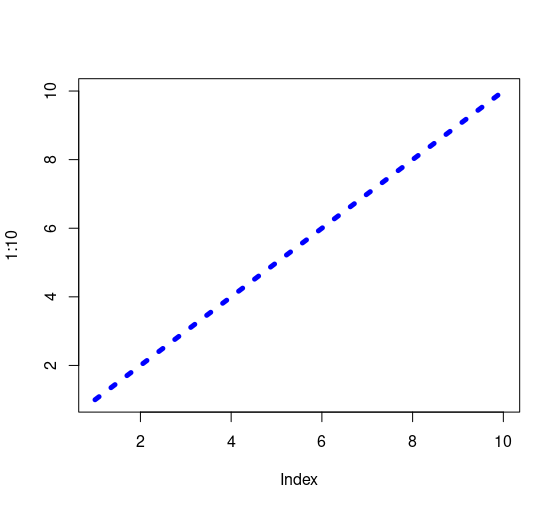• 0 删除该行（不显示）
• 1 显示实线（solid line）
• 2 显示虚线（dashed line）
• 3 显示点虚线（dotted line）
• 4 显示一条“点划线”（dot dashed line）
• 5 显示一条“长虚线”（long dashed line）
• 6 显示一条“双虚线”（two dashed line）

## 多线条

line1 <- c(1,2,3,4,5,10)
line2 <- c(2,5,7,8,9,10)

plot(line1, type = "l", col = "blue")
lines(line2, type="l", col = "red")